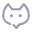PHP foreach &符号的用法，需要注意的坑

super
2021-04-16 12:261022

\$array = [
'a' => [
'a1' => 1,
'a2' => 2
],
'b' => [
'a1' => 1,
'a2' => 2
],
'c' => [
'a1' => 1,
'a2' => 2
]
];

foreach (\$array as \$k => \$v) {
\$array[\$k]['a1'] = 11;
}

foreach (\$array as \$k => &\$v) {
\$v['a1'] = 11;
}

unset(\$v); // \$v的值释放掉，这个很关键

foreach (\$array as \$k => &\$v) {
\$v['a2'] = 22;
}

0 条讨论